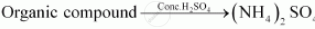Share

# Differentiate Between the Principle of Estimation of Nitrogen in an Organic Compound By Kjeldahl’S Method. - Chemistry

#### Question

Differentiate between the principle of estimation of nitrogen in an organic compound by Kjeldahl’s method.

#### Solution 1

In Kjeldahl’s method, a known quantity of nitrogen containing organic compound is heated with concentrated sulphuric acid. The nitrogen present in the compound is quantitatively converted into ammonium sulphate. It is then distilled with excess of sodium hydroxide. The ammonia evolved during this process is passed into a known volume of H2SO4. The chemical equations involved in the process are(NH_4)_2SO_4  + 2NaOH -> Na_2SO_4 + 2NH_3 + 2H_2O

2NH_3 + H_2SO_4 -> (NH_4)_2 SO_4

The acid that is left unused is estimated by volumetric analysis (titrating it against a standard alkali) and the amount of ammonia produced can be determined. Thus, the percentage of nitrogen in the compound can be estimated. This method cannot be applied to the compounds, in which nitrogen is present in a ring structure, and also not applicable to compounds containing nitro and azo groups.

#### Solution 2

Kjeldahl’s method: A known mass of the organic compound is heated strongly with cone. H2SO4, a little potassium sulphate and a little mercury (a catalyst). As a result of reaction the nitrogen present in the organic compound is converted to ammonium sulphate.

Is there an error in this question or solution?

#### APPEARS IN

NCERT Solution for Chemistry Textbook for Class 11 (2018 (Latest))
Chapter 12: Organic Chemistry - Some Basic Principles and Techniques
Q: 22.2 | Page no. 363
Solution Differentiate Between the Principle of Estimation of Nitrogen in an Organic Compound By Kjeldahl’S Method. Concept: Quantitative Analysis of Nitrogen.
S